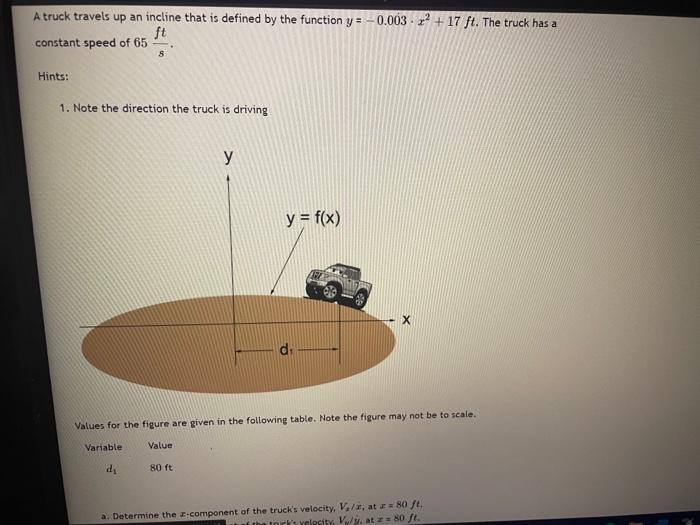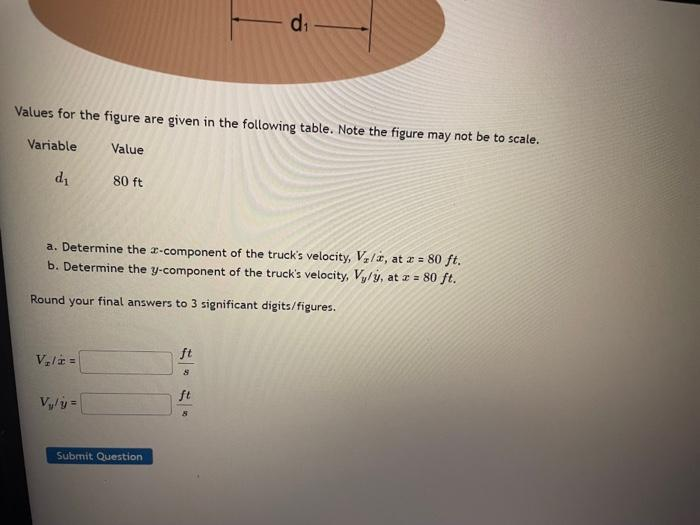# Question Solved1 AnswerA truck travels up an incline that is defined by the function y=-0.003x^2+17 ft. the truck has a constant speed of 65ft/s. Determine the x and y components velocities at x=80 ft A truck travels up an incline that is defined by the function y = -0.003 -x + 17 ft. The truck has a ft constant speed of 65 S Hints: 1. Note the direction the truck is driving у y = f(x) х Values for the figure are given in the following table. Note the figure may not be to scale. Variable Value di 80 ft a. Determine the r.component of the truck's velocity, V./, at 80 ft. the tor'synlecity, Vly at 80 l d: Values for the figure are given in the following table. Note the figure may not be to scale. Variable Value di 80 ft a. Determine the 1-component of the truck's velocity, V,14, at 2 = 80 ft. b. Determine the y-component of the truck's velocity, Vy/y, at I = 80 ft. Round your final answers to 3 significant digits/figures. ft V.lt S ft Vy- Submit QuestionA truck travels up an incline that is defined by the function y=-0.003x^2+17 ft. the truck has a constant speed of 65ft/s. Determine the x and y components velocities at x=80 ftTranscribed Image Text: A truck travels up an incline that is defined by the function y = -0.003 -x + 17 ft. The truck has a ft constant speed of 65 S Hints: 1. Note the direction the truck is driving у y = f(x) х Values for the figure are given in the following table. Note the figure may not be to scale. Variable Value di 80 ft a. Determine the r.component of the truck's velocity, V./, at 80 ft. the tor'synlecity, Vly at 80 l d: Values for the figure are given in the following table. Note the figure may not be to scale. Variable Value di 80 ft a. Determine the 1-component of the truck's velocity, V,14, at 2 = 80 ft. b. Determine the y-component of the truck's velocity, Vy/y, at I = 80 ft. Round your final answers to 3 significant digits/figures. ft V.lt S ft Vy- Submit Question
More
Transcribed Image Text: A truck travels up an incline that is defined by the function y = -0.003 -x + 17 ft. The truck has a ft constant speed of 65 S Hints: 1. Note the direction the truck is driving у y = f(x) х Values for the figure are given in the following table. Note the figure may not be to scale. Variable Value di 80 ft a. Determine the r.component of the truck's velocity, V./, at 80 ft. the tor'synlecity, Vly at 80 l d: Values for the figure are given in the following table. Note the figure may not be to scale. Variable Value di 80 ft a. Determine the 1-component of the truck's velocity, V,14, at 2 = 80 ft. b. Determine the y-component of the truck's velocity, Vy/y, at I = 80 ft. Round your final answers to 3 significant digits/figures. ft V.lt S ft Vy- Submit Question